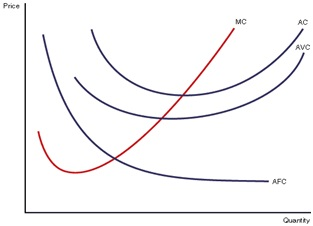# Average Cost

Posted in Operations and Supply Chain Terms, Total Reads: 1826

## Definition: Average Cost

As the name suggests, it is average of total cost (TC) over the quantity produced or ordered. In economics, total cost comprises of total variable cost (TVC) and total fixed cost (TFC). Hence, average cost (AC) can be written as,

AC= TC/Q=(TVC+TFC)/Q=AVC+AFC

Here, AVC and AFC are average variable and fixed cost respectively.

In case of ordering, total cost consists of ordering cost, purchasing cost and inventory holding cost that can be divided by ordered quantity to get average cost.The graph depicts how AC, MC (Marginal Cost) and AVC vary in a U shaped curve. For smaller quantities, the difference between AVC and AVC is more in comparison to difference between them for larger quantities. It happens because of decrease in average fixed cost with quantity. For very large quantity, AC and AVC become approximately equal. Also, it’s interesting to note that MC intersects AC and AVC at their minimum point.

Example: Suppose you have a plant with a fixed cost of Rs 1 million that produces 1 million bottles at a variable cost of Rs 2/bottle. The total cost will be 3 million for producing 1 million bottles. Hence, Average cost will be Rs 3 per bottle. It’s also important to note that you can’t decrease average cost beyond a particular quantity as average variable cost starts increasing after a limit.

Hence, this concludes the definition of Average Cost along with its overview.

Browse the definition and meaning of more terms similar to Average Cost. The Management Dictionary covers over 7000 business concepts from 6 categories.

Search & Explore : Management Dictionary

Similar Definitions from same Category: